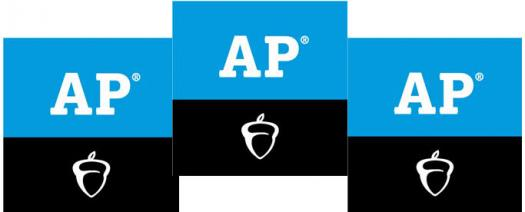# The Ultimate AP Calculus Quiz!

10 Questions | Total Attempts: 105SettingsAdvanced Placement Calculus is a set of two distinct Advanced Placement calculus courses and exams offered by College Board. AP Calculus AB covers limits, derivatives, and integrals. AP Calculus BC covers all AP Calculus AB topics plus additional topics (including more integration techniques such as integration by parts, Taylor series, parametric equations, polar coordinate functions, and curve interpolations). The test is not the actual AP. This, prepares you for the actual test.

• 1.
If a and b are integers such that 4a – 8 > 0 and 4b + 8 < 0, then which of the following must be true?
• A.

Ab is even

• B.

Ab is odd

• C.

Ab is negative

• D.

Ab is positive

• 2.
If z is a positive number not equal to 1, which of the following must also be positive?
• A.

B/b+1

• B.

B+6/b-3

• C.

1/2b-2

• D.

2-b

• 3.
For all x, let f(x) = (10 – x)^2. If y = f(6), which of the following is equal to 4y?
• A.

F(24)

• B.

F(18)

• C.

F(12)

• D.

F(8)

• 4.
What is the distance between the b-intercept and the y-intercept of the line c = 2/3x – 6?
• A.

9

• B.

15

• C.

√89

• D.

√117

• 5.
Which of the following is equal to 3a + 9b + 12?
• A.

3(a + 3b + 9)

• B.

3(a + 3b)+12

• C.

3(a + 3b)+4

• D.

3(a + 6b + 9)

• 6.
If f(x) = √3x – 2, what is the smallest possible value of f(x)?
• A.

0

• B.

2/3

• C.

4

• D.

1

• 7.
If x​​​​​​2 – |5x| = –6, then what is one possible value of x?
• A.

6

• B.

2

• C.

0

• D.

5

• 8.
If 3(a – 2) = 24, then what does 5(a – 2) equal?
• A.

35

• B.

43

• C.

40

• D.

27

• 9.
If √a + 22 = 38, a =
• A.

234

• B.

256

• C.

125

• D.

250

• 10.
If b is an integer between 50 and 70 and can be expressed as 7z + 3 where z is an integer, what are the possible values of b?
• A.

50,59,66

• B.

52,59,66

• C.

52,58,66

• D.

52,59,65

Related TopicsBack to top